Updating search results...

# 10 Results

View
Selected filters:
• CCSS.Math.Content.6.EE.B.8Only Sharing Permitted
CC BY-NC-ND
Rating
4.0 stars

This lesson unit is intended to help you assess how well students are able to: Perform arithmetic operations, including those involving whole-number exponents, recognizing and applying the conventional order of operations; Write and evaluate numerical expressions from diagrammatic representations and be able to identify equivalent expressions; apply the distributive and commutative properties appropriately; and use the method for finding areas of compound rectangles.

Subject:
Geometry
Material Type:
Assessment
Lesson Plan
Provider:
Shell Center for Mathematical Education
Provider Set:
Mathematics Assessment Project (MAP)
04/26/2013Conditional Remix & Share Permitted
CC BY-NC
Rating
0.0 stars

Subject:
Mathematics
Material Type:
Full Course
Provider:
Pearson
10/06/2016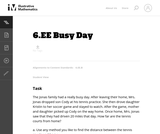Unrestricted Use
CC BY
Rating
3.0 stars

This task provides a good entry point for students into representing quantities in contexts with variables and expressions and building equations that reflect the relationships presented in the context.

Subject:
Mathematics
Material Type:
Activity/Lab
Provider:
Illustrative Mathematics
Provider Set:
Illustrative Mathematics
Author:
Illustrative Mathematics
08/15/2012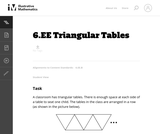Unrestricted Use
CC BY
Rating
0.0 stars

This task provides a good opportunity for group work and class discussions where students generate and compare equivalent expressions. In class discussion, students should be asked to connect the terms of an expression with quantities shown in the diagram.

Subject:
Mathematics
Material Type:
Activity/Lab
Provider:
Illustrative Mathematics
Provider Set:
Illustrative Mathematics
Author:
Illustrative Mathematics
05/01/2012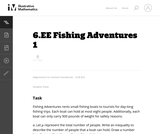Unrestricted Use
CC BY
Rating
0.0 stars

This task is the first in a series of three tasks that use inequalities in the same context at increasing complexity in 6th grade, 7th grade and in HS algebra. Students write and solve inequalities, and represent the solutions graphically.

Subject:
Mathematics
Material Type:
Activity/Lab
Provider:
Illustrative Mathematics
Provider Set:
Illustrative Mathematics
Author:
Illustrative Mathematics
05/01/2012Conditional Remix & Share Permitted
CC BY-NC
Rating
0.0 stars

Students work in pairs to critique and improve their work on the Self Check from the previous lesson.Key ConceptsTo critique and improve the task from the Self Check and to complete a similar task with a partner, students use what they know about solving equations and relating the equations to real-world situations.Goals and Learning ObjectivesSolve equations using the addition or multiplication property of equality.Write word problems that match algebraic equations.Write equations to represent a mathematical situation.

Subject:
Algebra
Material Type:
Lesson Plan
Provider:
Pearson
09/21/2015Conditional Remix & Share Permitted
CC BY-NC
Rating
0.0 stars

Lesson OverviewStudents represent real-world situations using inequality statements that include a variable.Key ConceptsInequality statements tell you whether values in a situation are greater than or less than a given number and also tell you whether values in the situation can be equal to that number or not.The symbols < and > tell you that the unknown value(s) in a situation cannot be equal to a given number: the unknown value(s) are strictly greater than or less than the number. The inequality x < y means x must be less than y. The inequality x > y means x must be greater than y.The symbols ≤  and ≥ tell you that the unknown value(s) in a situation can also be equal to a given number: the unknown value(s) are less than or equal to, or greater than or equal to, the number. The inequality x ≤ y means x is less than or equal to y. The inequality x ≥ y means x is greater than or equal to y.Goals and Learning ObjectivesUnderstand the inequality symbols <, >, ≤, and ≥.Write inequality statements for real-world situations.ELL: When writing the summary, provide ELLs access to a dictionary and give them time to discuss their summary with a partner before writing, to help them organize their thoughts. Allow ELLs who share the same primary language to discuss in their native language if they wish.

Subject:
Numbers and Operations
Material Type:
Lesson Plan
Provider:
Pearson
09/21/2015Conditional Remix & Share Permitted
CC BY-NC
Rating
0.0 stars

Lesson OverviewStudents represent inequalities on a number line, find at least one value that makes the inequality true, and write the inequality using words.SWD:When calling on students, be sure to call on ELLs and to encourage them to actively participate. Understand that their pace might be slower or they might be shy or more reluctant to volunteer due to their weaker command of the language.SWD:Thinking aloud is one strategy for making learning visible. When teachers think aloud, they are externalizing their internal thought processes. Doing so may provide students with insights into mathematical thinking and ways of tackling problems. It also helps to model accurate mathematical language.Key ConceptsInequalities, like equations, have solutions. An arrow on the number line—pointing to the right for greater values and to the left for lesser values—can be used to show that there are infinitely many solutions to an inequality.The solutions to x < a are represented on the number line by an arrow pointing to the left from an open circle at a.Example: x < 2The solutions to x > a are represented on the number line with an arrow pointing to the right from an open circle at a.Example: x > 2The solutions to x ≤ a are represented on the number line with an arrow pointing to the left from a closed circle at a.Example: x ≤ 2The solutions to x ≥ a are represented on the number line with an arrow pointing to the right from a closed circle at a.Example: x ≥ 2Goals and Learning ObjectivesRepresent an inequality on a number line and using words.Understand that inequalities have infinitely many solutions.

Subject:
Numbers and Operations
Material Type:
Lesson Plan
Provider:
Pearson
09/21/2015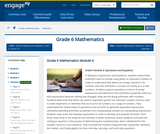Conditional Remix & Share Permitted
CC BY-NC-SA
Rating
0.0 stars

In Module 4, Expressions and Equations, students extend their arithmetic work to include using letters to represent numbers in order to understand that letters are simply "stand-ins" for numbers and that arithmetic is carried out exactly as it is with numbers. Students explore operations in terms of verbal expressions and determine that arithmetic properties hold true with expressions because nothing has changedthey are still doing arithmetic with numbers. Students determine that letters are used to represent specific but unknown numbers and are used to make statements or identities that are true for all numbers or a range of numbers. They understand the relationships of operations and use them to generate equivalent expressions, ultimately extending arithmetic properties from manipulating numbers to manipulating expressions. Students read, write and evaluate expressions in order to develop and evaluate formulas. From there, they move to the study of true and false number sentences, where students conclude that solving an equation is the process of determining the number(s) that, when substituted for the variable, result in a true sentence. They conclude the module using arithmetic properties, identities, bar models, and finally algebra to solve one-step, two-step, and multi-step equations.

Subject:
Numbers and Operations
Material Type:
Module
Provider:
New York State Education Department
Provider Set:
EngageNY
12/17/2013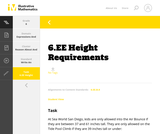Unrestricted Use
CC BY
Rating
0.0 stars

This is a task from the Illustrative Mathematics website that is one part of a complete illustration of the standard to which it is aligned. Each task has at least one solution and some commentary that addresses important aspects of the task and its potential use.

Subject:
Numbers and Operations
Material Type:
Activity/Lab
Provider:
Illustrative Mathematics
Provider Set:
Illustrative Mathematics
Author:
Illustrative Mathematics